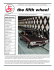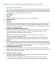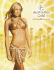# Snakeboard Motion Planning With Local Trajectory Information

## Transcription

Snakeboard Motion Planning With Local Trajectory Information
```Proceedings of the ASME 2013 Dynamic Systems and Control Conference
DSCC2013
October 21-23, 2013, Palo Alto, California, USA
DSCC2013-3883
SNAKEBOARD MOTION PLANNING WITH LOCAL TRAJECTORY INFORMATION
Tony Dear
Robotics Institute
Carnegie Mellon University
Pittsburgh, Pennsylvania 15232
[email protected]
Ross L. Hatton
School of Mechanical, Industrial,
and Manufacturing Engineering
Oregon State University
Corvallis, Oregon 97331
[email protected]
ABSTRACT
We address trajectory generation for the snakeboard, a system commonly studied in the geometric mechanics community.
Our approach derives a solution using body coordinates and local trajectory information, leading to a more intuitive solution
compared to prior work. The simple forms of the solution clearly
show how they depend on local curvature and desired velocity
profile, allowing for a description of some simple motion primitives. We readily propose techniques to navigate paths, including those with sharp corners, by taking advantage of the snakeboard’s singular configuration, as well as discuss some implications of torque limits.
Figure 1.
Matthew Travers
Howie Choset
Robotics Institute
Carnegie Mellon University
Pittsburgh, Pennsylvania 15232
[email protected],
[email protected]
A SNAKEBOARD COMPOSED OF A RIGID AXIS AND TWO
FOOTRESTS ON ROTATING WHEELSETS.
tem to progress along a trajectory despite joint limits. Ostrowski
et al.  used techniques developed by Murray and Sastry  to
evaluate sinusoidal steering controllers to move the snakeboard
in various basis directions. Ostrowski et al.  then extended
this approach by using optimal control to select optimal gaits for
their controllers.
Bullo and Lewis  addressed the motion planning problem of taking a snakeboard to a desired goal state from a given
start state by using vector field analysis techniques developed
by Bullo et al. . In these solutions, the snakeboard’s overall trajectory was actually a concatenation of separate kinematic
trajectory segments between the start and goal states.
In order to study the contributions of gaits to locomotion,
Shammas et al.  analyzed gait motions as a combination of
geometric and dynamic displacement contributions. They were
able to propose gaits that contribute to motion along a desired
direction, although their method did not address the full motion
planning problem of finding a trajectory between two states.
1
INTRODUCTION
The snakeboard is a canonical example of a mixed nonholonomic mechanical system, one whose motion is governed
by both kinematic constraints and dynamics [1, 2]. The snakeboard shown in Fig. 1 consists of two sets of wheels that can
rotate about the center point of the axle. To ride the snakeboard,
one alternates between rotating one’s torso with one’s ankles to
move the wheelsets. The mechanical model in Fig. 2 has a rotor
situated at the center of the longitudinal axis to simulate the human rider’s torso. The rotor and wheel angles are actuated, and
the latter act as constraints, allowing the snakeboard to locomote
when its joint angles are changing.
Early approaches in snakeboard analysis employed extensive use of gaits, or cyclic changes in the joint inputs. A key
feature of using gaits for locomotion is that they allow for a sys-
1
are related to the world velocities by the following mapping2 .
  
ξx
cos θ sin θ
ξ = ξy  =  − sin θ cos θ
ξθ
0
0
Figure 2.
 
ẋ
0
0  ẏ 
1
θ̇
(1)
The snakeboard’s mass and inertia are denoted M and J,
while the rotor and wheel inertias are denoted Jr , and Jw , respectively. We will assume as in  that ML2 = J + Jr + 2Jw
is the total inertia of the system, where the total length of the
snakeboard is 2L.
The snakeboard’s Lagrangian, which is simply its kinetic
energy, is invariant to changes in the system’s position or orientation in space, so we can express it in body velocities instead
of world velocities. In the absence of constraints, this property
is equivalent to an inherent symmetry of the system; the fact that
the dynamics are unchanged with respect to rigid transformations
of the body frame corresponds to momentum conservation. The
Lagrangian in the body frame is
THE CONFIGURATION OF THE SNAKEBOARD.
More recently, Shammas and de Oliveira [8, 9] developed a
solution for the snakeboard in which the problem specification is
an entire trajectory, not just the endpoint configurations. Their
approach assumes that the joint motions are unconstrained and
thus do not require gaits, in contrast to previous work. Without
the restriction of gaits, they are able to analytically solve for the
joint angles in order to follow the prescribed trajectory.
In this paper, we build upon the results of [8, 9] and propose a simpler representation of the solution using only body
coordinates and local curvature information of the desired path.
We have found that this approach yields much insight into the
properties and limits of the control problem. This solution also
presents us with simple primitives that can be stitched together to
form more complex trajectories. Finally, we comment on some
types of the paths that the snakeboard can or cannot follow and
show some simulation results of the derived solutions.
l(ξ, ṙ) =
M(ξ2x + ξ2y + L2 ξ2θ ) + Jr (2ξθ ψ̇ + ψ̇2 ) + 2Jw φ̇2
.
2
(2)
The no slip conditions on the wheels give rise to the system’s
nonholonomic constraints. Like the Lagrangian, the constraints
are invariant with respect to transformations in SE(2), so they
can be written in terms of the local body coordinates only. They
can be expressed in Pfaffian form ω(r)ξ = 0, where
ω(r) =
EQUATIONS OF MOTION
The snakeboard’s configuration, shown in Fig. 2, is given by
q ∈ Q = (g, r), where the position variables g = (x, y, θ) ∈ G =
SE(2) locate the snakeboard in the world and the shape variables
r = (ψ, φ) ∈ M = S1 × S1 describe the system’s joint configurations.1 The variables x and y denote the global position of the
snakeboard’s center of mass, while θ denotes the system’s longitudinal axis orientation with respect to the horizontal. ψ denotes
the rotor angle, and φ f and φb denote the angles of the front and
back sets of wheels, respectively, both with respect to the longitudinal axis. We enforce the constraint φ f = −φb and henceforth
use φ = φ f , which will simplify the problem while still allowing
for a full solution.
The world velocity of the system is ġ = (ẋ, ẏ, θ̇). However, in
this paper, we work with the body velocities ξ instead, which has
components in the forward, lateral, and rotation directions. They
− sin φ
sin φ
cos φ L cos φ
.
cos φ −L cos φ
(3)
2
Given the Lagrangian and constraints, it is possible to completely determine the evolution of the system through the EulerLagrange equations of motion, i.e.,
2
∂l(ξ, ṙ)
j
+ ∑ λ j ωi (r) = 0, i = 1, 2, 3
∂ξi
j=1
d ∂l(ξ, ṙ)
d ∂l(ξ, ṙ)
= τψ ,
= τφ .
dt
∂ψ̇
dt
∂φ̇
d
dt
(4)
Here, i indexes the body coordinate, the Lagrange multiplier for
the jth constraint is λ j , and the input torques for the rotor and
wheel sets are τψ and τφ , respectively.
2 G = SE(2) is a Lie group, whose tangent space is equipped with the lifted
left action corresponding to the mapping above. The body velocity ξ lives in the
Lie algebra g, which is homeomorphic to the tangent space at the identity of the
group. For further reading on the topic, see  and .
1 In the context of geometric mechanics, Q = M × G is a trivial principal fiber
bundle, M is the base space, and G is the fiber space.
2
However, the Euler-Lagrange equations do not allow an
easy solution for the inverse motion planning problem. Given a
planned trajectory, it is difficult to invert the equations of motion
to derive the input forces and torques required to follow it. Similar to previous work, the approach in this paper uses tools from
geometric mechanics for an alternate formulation of the problem.
From Eq. (2) and Eq. (3) we first compute the nonholonomic
∂l T
Ω , where ΩT is a
momentum  using the definition p = ∂ξ
basis of the null space of ω. The nonholonomic momentum thus
∂l
projects the generalized momentum ∂ξ
onto a basis that respects
the constraints. We choose Ω = L, 0, tan φ and find
p = ML(ξx + Lξθ tan φ) + Jr ψ̇ tan φ.
The curvature κ(s) is positive if the unit tangent vector along the
curve rotates in a counter-clockwise direction as a function of
arclength; otherwise, it is negative. We assume that we are given
initial conditions that describe the starting location on the curve.
The distance r(t) tells us how much distance to travel over
time. Note that it is possible to go backward if ṙ < 0. Since the
arclength is equivalent to distance traveled, we have s = r(t). In
general, we also require that r be twice-differentiable and κ be
once-differentiable. Then the function
c(t) := κ(s) = κ(r(t))
(5)
is a differentiable path curvature function parameterized in time.
We wish to solve for the shape trajectory φ(t) and shape velocity ψ̇(t) such that the x-axis of the local body frame is tangent
to the curve described by c(t), and the total distance traveled
along the curve by the snakeboard’s center of mass is equal to
r(t). Note that we do not have to solve for ψ(t) explicitly, since it
does not appear in any of the equations of motion. Physically, the
orientation of the rotor does not matter due to rotational symmetry; only changes in its velocity contribute to the overall system
dynamics.
This method of path parameterization is different from that
of , where the path is paramerized using world coordinates,
namely x and y. Although that approach explicitly provides the
center of mass location at any given time, it forces the adoption
of a fixed inertial frame. This in turn significantly increases the
complexity of the solution with the use of intermediary variables.
Next we examine how joint velocities and and momentum
determine resultant body velocities. Combining the constraints
Eq. (3) and the momentum definition Eq. (5), we find the following system of equations:3


ξ = −
Jr sin 2φ
2ML
0
Jr sin2 φ
ML2
 2 

cos φ
0 ψ̇
 ML 
0
+  0  p.

φ̇
sin 2φ
0
2ML2
(6)
Finally, we solve for the momentum evolution equation ,
which describes how the momentum changes in time. This can
be derived by substituting Eq. (6) into the Euler-Lagrange equations of motion Eq. (4) for the body velocities. One can then
eliminate the Lagrange multipliers and solve for ṗ to obtain
ṗ = φ̇(p tan φ + Jr ψ̇).
(10)
(7)
4
THE SNAKEBOARD SOLUTION
Given the problem specification, we can now explicitly
solve for the joint angles that will allow the snakeboard to adhere to the desired curvature and arclength functions. We follow
a procedure similar to that of , but the resultant solution is
much simpler.
3
THE MOTION PLANNING PROBLEM
The motion planning problem for the snakeboard is to take
a specified path between a start and a goal location in space, and
determine a trajectory for the rotor and wheel angles that propels
the snakeboard along the path. The path to be followed is a curve
in space, which can be defined by its curvature as a function of
its arclength, along with initial conditions. Since the snakeboard
is moving along the curve in time, we also dictate the amount of
distance to be traveled as a function of time.
We formulate the problem as follows. The spatial curve to
be followed will be parameterized in s and defined in terms of
curvature. We define κ(s) and r(t) to be the curvature and desired
distance functions, respectively, where
κ : R → R,
r : [0, T ] → R.
4.1
The Joint Angles
We first obtain a solution for the wheel angles φ(t) by examining the geometry of the snakeboard on the trajectory at any
given point in time (Fig. 3). We have that the longitudinal axis
is instantaneously tangent to the path at the point P. Then the
snakeboard’s longitudinal axis must be orthogonal to the curvature radius R, which is simply the inverse of the curvature itself.
By trigonometry, the angle between the front wheels’ axis
and the radius of curvature is equal to φ. Thus, the wheel angles
and the snakeboard’s steering profile are completely determined
by the local curvature of the path to be followed:
(8)
(9)
φ(t) = φ f (t) = tan−1 (Lc(t)) = −φb (t).
3 This is the reconstruction equation, which has the form ξ = −A(r)ṙ + Γ(r)p.
3
(11)
The tangency requirement also requires that the snakeboard’s forward velocity be equal to the rate of change in arclength, ṙ(t). Since r(t) and thus ṙ(t) are provided, we can solve
the remainder of the problem assuming that ξx is known:
ξx (t) = ṙ(t).
(12)
We can also solve for the y and θ components of the body
velocity. They are completely specified by the constraints Eq.
(3), since we now know the wheel angles φ(t):
ξy (t) = 0,
(13)
tan φ(t)
ξθ (t) =
ξx (t) = c(t)ṙ(t).
L
(14)
Figure 3.
The other shape input to be determined is the rotor velocity,
which controls the forward velocity of the snakeboard. We differentiate the momentum Eq. (5) to obtain an expression for ṗ.
This can then be equated to the momentum evolution Eq. (7):
4.2.1 Constant Forward Velocity. For constant forward velocity, arclength is increasing at a constant rate; ṙ is constant and r̈ = 0. If t0 is the time at which the velocity becomes
constant, then the rotor velocity has the following solution:
ṗ = ML(ξ˙ x + Lξ˙ θ tan φ + Lξθ φ̇ sec2 φ) + Jr (ψ̇φ̇ sec2 φ + ψ̈ tan φ)
= MLφ̇(ξx tan φ + Lξθ tan2 φ) + Jr φ̇(ψ̇ tan2 φ + ψ̇).
ψ̇(t) = −
(15)
2ML ˙
ξx − MLφ̇ sec2 φξx
sin 2φ
Z t
1 + L2 c(τ)2
0
c(τ)
ML2
r̈(τ) dτ −
Jr
(18)
4.2.2 Zero Curvature. When the curvature κ = 0, the
path becomes straight, and both the front and back sets of wheels
are aligned with the path, i.e.,
(16)
If we integrate this equation once, we obtain a solution for
ψ̇ as a definite integral of the path parameterization functions:
M
ψ̇(t) = −
Jr
ML2 c(t) − c(t0 ) ṙ + ψ̇(t0 ).
Jr
For a general trajectory in which the goal is to simply maintain a
constant forward velocity, the change in rotor velocity thus varies
linearly with the curvature of the path to be followed.
By substituting the expression for ξθ from Eq. (14) into Eq.
(15), we can obtain a second-order differential equation for ψ,
which is solvable in the known quantities.
Jr ψ̈ = −
THE GEOMETRY OF THE SNAKEBOARD’S TRAJECTORY.
φ(t) = 0.
(19)
In this configuration, ξy = ξθ = 0, but there is no prescription
for ξx . This results in a symmetry in the longitudinal direction,
which corresponds to the conservation of linear momentum. The
snakeboard must enter and leave this regime with the same momentum, implying that the forward velocity remains constant.
Because of the invariance in forward velocity, the rotor has
no effect on the motion of the snakeboard, and so its orientation
and velocity can be reset arbitrarily during the period in which
the curvature remains 0. This can be a useful technique for preventing the rotor’s velocity from becoming too large.
Z t
ċ(τ)ṙ(τ) dτ. (17)
0
4.2
Special Cases
From the solutions to the shape variables of the snakeboard,
some simple primitives governing its motion can be inferred
for special cases of curvature and forward speed. We will also
discuss a method for dealing with non-differentiable paths, for
which Eq. (17) does not have a solution. This case was not addressed by the solution of .
4.2.3 Constant, Nonzero Curvature. For constant,
nonzero curvature c = c0 , Eq. (11) again yields a constant wheel
4
angle solution:
φ(t) = φ0 = tan−1 (Lc0 ).
(20)
Thus φ̇ = 0, and from Eq. (7) we can also conclude that ṗ = 0.
The total momentum must be conserved such that p = p0 during
the interval on which the snakeboard moves along the constant
curvature section, where p0 is the momentum built up by the
system at the time that it enters this section.
If t0 is the time at which the curvature becomes constant,
then the rotor velocity profile is given as follows:
M
ψ̇(t) = −
Jr
1 + L2 c20
c0
Figure 4.
(ṙ(t) − ṙ(t0 )) + ψ̇(t0 ).
Since corners may appear in a physical trajectory, we propose the following technique to traverse them. The snakeboard
must be at rest before and after turning the corner, so we require that a corner occurs at the intersection of two curves with
nonzero curvature. Thus, the forward velocity ṙ must encode the
corner with zero forward velocity.
The snakeboard first steers into the corner by following the
path curvature and then stops completely by spinning the rotor
according to Eq. (17). Next the wheel angles will turn to achieve
φ = π2 . From Eq. (23), we can use the rotor to make the snakeboard spin in place until its axis is tangent to the new path segment. In the last step, we rotate the wheel axes to match the new
curvature and resume rotor movement to continue moving again.
The described maneuver is equivalent to restarting the
snakeboard from a resting configuration. The initial condition
of the new segment’s orientation is encoded in the magnitude of
the impulse function, which measures the change in orientation
between the two path segments.
(21)
The rotor velocity varies linearly with the desired forward velocity for a constant curvature path. This is analogous to Eq. (18),
the case of constant forward velocity and changing curvature.
4.2.4 The Singular Configuration. When the path
curvature goes to infinity, as at a sharp corner, the path becomes
non-differentiable. The solution for the wheel angles is
π
φ(t) = ± .
2
(22)
The wheels are oriented at right angles to the snakeboard’s axis,
and so the system can only turn in place while being unable to
move forward. Our previous stipulations for the body velocities
are invalid for this singular configuration, as we now have that
ξx = 0 and Eq. (14) no longer holds.
However, we can relate the rotor angular velocity to that of
the snakeboard. Substituting φ(t) = ± π2 into Eq. (6) gives us
ξθ = −
Jr
ψ̇(t) + ψ̇(t0 ),
ML2
AVOIDING SOME OBSTACLES VIA A SHARP CORNER.
4.3
Allowed and Prohibited Trajectories
We now consider the effect of torque limits on the snakeboard’s ability to handle trajectories, as our solution assumes that
joint angles can accelerate arbitrarily quickly, which may be nonphysical. We emphasize that we can easily interpret the physical
meanings of such limits due to the simple forms of our solutions.
Limits on the wheel torques τφ will limit how fast the snakeboard can change direction. If the wheels’ maximum acceleration is φ̈m , then from the second derivative of Eq. (11) the curvature function c must satisfy
(23)
where t0 is the time at which the snakeboard achieves the singular
configuration. This is simply a statement of the conservation
of angular momentum about the snakeboard’s center of rotation,
where the momentum comes from the rotor velocity at t0 .
As shown in Fig. 4, infinite curvature can occur discontinuously at a sharp corner in the path. At this location, c is instantaneously characterized by an impulse function, and we cannot use
Eq. (17) to solve for the inverse kinematics of the joint angles.
Physically, an infinite amount of force is required to instantaneously change the wheel angles in a discontinuous manner if
the snakeboard’s forward velocity is nonzero.
˙2 1
≤ φ̈m ,
¨c̃ − 2c̃c̃
1 + c̃2
1 + c̃2 (24)
where we define the scaled curvature c̃ = Lc. If this inequality
does not hold, then the snakeboard will be unable to adhere to
the prescribed path cuvature.
5
We can interpret Eq. (24) as a limit on the sharpness of turns
that the snakeboard can make. An example curvature characteristic of a sharp turn is shown in Fig. 5, along with its derivatives.
Notice that the zero of c̃˙ coincides with an extremum of c̃¨, while
the extrema of c̃˙ coincide with the zeros of c̃¨.
At these points, the left-hand side of Eq. (24) becomes large,
and the magnitudes of c̃˙ and c̃¨ scale with the sharpness of the
turn. In the limit as c → ∞ and tends toward an impulse function,
such turns will become sharp corners, and the the snakeboard
will have to use the singular configuration to navigate them.
If we limit the torque applied at the rotor, then the rotor’s
maximum acceleration will be bounded by some ψ̈m . From Eq.
(16), the following inequality must be satisfied:
ML 2ξ˙ x
2
+ φ̇ sec φξx ≤ ψ̈m .
Jr sin 2φ
Time
(25)
Figure 5.
The first term on the left-hand side of Eq. (25) limits the forward
acceleration of the snakeboard, especially when the wheel angles
are near 0, π2 , and π. This is sensible, as we have seen that it
becomes increasingly difficult for the snakeboard to control its
forward velocity near these configurations.
The second term limits the forward velocity ξx itself. As
φ → π2 , the snakeboard approaches its singular configuration, and
the forward velocity ξx must decrease to prevent the term from
becoming unbounded. In addition, the presence of φ̇ restricts
the system from simultaneously traveling forward and changing
directions too quickly.
In general, all of these joint limits prevent the snakeboard
from turning arbitrarily quickly around a point of high curvature.
The tighter the turn that the system has to make, the more it must
slow down to do so without going off the prescribed path.
THE CURVATURE PROFILES OF A SHARP TURN.
shown in Table 3 of , which ensures smoothness of first
derivatives. In addition, these polynomial curves can be integrated explicitly, so that we can obtain r(t) if ṙ(t) is described by
a transition curve.
Unless otherwise stated, we use the initial conditions
(x0 , y0 , θ0 ) = (0, 0, 0) for all simulations. We use the same
snakeboard parameters as those in :
M = 4,
Jr = 2,
1
Jw = ,
2
J = 1,
L = 1.
5.1
Constant Velocity Curves
We first consider the problem of maintaining constant velocity over turns with maximum curvature c0 = κ0 . All turns start
and end at arclengths of s1 and s2 , respectively. The curvature
κ is 0 for s < s1 and s > s2 , and the two disjoint zero segments
are smoothly connected by increasing and decreasing transition
curves of varying magnitudes.
We simulate three trajectories with c0 = 0.1, 0.2, and 0.3.
For the distance function, we choose r(t) = t such that the forward velocity is constant. Thus, the rotor profile is easily determined by (18), while the wheel angle comes from Eq. (11).
The snakeboard’s resultant trajectories, along with snapshots at equal time intervals, is shown in Fig. 6. The system
follows all curves with constant velocity, as expected. Note that
the larger the maximum curvature, the greater the turn achieved.
5
SIMULATIONS
We will now apply the general control solutions of Eq. (11)
and Eq. (17) for the joint angles to some specific trajectory examples. In doing so, we will also refer to the special case results
in Section 4.2 to analyze path feasibility and to characterize the
system behavior.
As a motivating example, we will make use of track transition curves from civil engineering to construct trajectory profiles.
Track transition curves smoothly connect straight and curved
sections of roads and railway tracks [13, 14]. Such curves are
usually formulated as higher-order polynomials that ensure that
derivatives along the track remain continuous, thus minimizing
changes in lateral acceleration to the locomoting system.
The path curvatures, as well as the desired velocities, that we
command in the following examples consist mainly of straight
sections connected by transition curve sections. We will prescribe the transition curvatures by the “fifth-order parabola” as
5.2
Accelerating Over Nonzero Curvature
We have seen that the snakeboard cannot change its forward velocity while traveling straight. One way that the system
can circumvent this is to temporarily deviate from its path on a
curved segment, accelerate, and then resume its original path.
6
10
25
y(t)
20
5
0
15
y(t)
−5
−10
0
10
20
30
40
50
x(t)
10
Figure 8.
5
ACCELERATING ON CONSTANT CURVATURE.
0
0
5
10
15
20
x(t)
25
30
35
40
35
Figure 6.
CONSTANT VELOCITY TRAJECTORIES.
30
25
20
3
15
2
10
1
5
0
0
−1
0
10
20
30
40
50
Time
−2
−3
Figure 9.
−4
TRAJECTORY PROFILE WITH A CORNER.
−5
−6
−7
0
10
20
30
40
5.3
A Trajectory with Corners
For the last example, we demonstrate an example trajectory
with a sharp corner. We have shown that one way of maneuvering a corner is equivalent to stopping, reorienting the system, and
then moving again. Figure 9 shows both the curvature c(t) and
desired distance function r(t).
The period during which c(t) is infinite and r(t) is constant
exactly corresponds to when the snakeboard is at the corner. The
traveled distance r should not change while the snakeboard is not
moving forward, while c is described by an impulse function.
Figure 10 shows the wheel angles and orientation of the
snakeboard. Note the ordering of the steps in the turning maneuver: φ first goes to π2 , and then θ aligns with the new path by
rotation action of the rotor. When that is complete, φ turns to its
new angle, and then the system again resumes its locomotion.
50
Time
Figure 7.
TRAJECTORY AND ROTOR INPUT PROFILES.
Here we will accelerate on a constant curvature only, using the
analytical result of Eq. (21).
As shown in Fig. 7, the curvature c(t) has three segments of
constant curvature connected by two transition curves. The desired velocity ṙ(t) is constant nearly everywhere, and its transition curve occurs in the regime where c is constant and nonzero.
From these functions we can find ψ̇(t), using both Eq. (18) and
Eq. (21).
Figure 8 shows the trajectory of the snakeboard, which executes the maneuver described by doubling its velocity after the
curved section. We also superimpose the rotor velocity ψ̇(t) on
top of the trajectory profiles in Fig. 7. Note how the rotor must
accelerate to either maintain the system’s constant forward velocity while path curvature is changing, or to change forward
velocity on a constant curvature path.
6
CONCLUSIONS
We have presented explicit solutions for the inverse motion
planning problem for the snakeboard. In doing so, we rely only
on local trajectory information of curvature and arclength, and
the solution is simple and easy to analyze due to our choice of
body coordinates. We take advantage of their forms to derive
7
1.6

1.4
1.2
1
0.8
0.6

0.4
0.2
0
−0.2
0
10
20
30
40
50

Time
Figure 10.
WHEELS AND ROTOR DURING A CORNER MANEUVER.

20
15

10
y(t)
5

0
−5
−5
0
5
10
15
20
25
x(t)

Figure 11.
MANEUVERING A CORNER.

motion primitives, as well as a method for dealing with nondifferentiable paths. Finally, we discussed practical limitations
that may be taken into account for a real system.
Since we now know what types of trajectories the snakeboard can and cannot follow, future work may revisit the problem in which only the initial and goal states are provided. These
results can be combined with the latest work in path planning in
order to construct efficient paths that are easy to follow, especially paths that consist mainly of constant curvature sections.
Another interesting direction would be to investigate how
much the usage of body coordinates generalizes in simplifying
solutions for dynamical systems. While they can present simpler
solutions than their inertial coordinate counterparts, using them
to move back into the world frame to compute various quantities
can often be a difficult or computationally expensive problem.





REFERENCES
 Ostrowski, J., Burdick, J., Lewis, A., and Murray, R., 1995.
“The mechanics of undulatory locomotion: the mixed kinematic and dynamic case”. In Robotics and Automation,
8
1995. Proceedings., 1995 IEEE International Conference
on, Vol. 2, pp. 1945 –1951 vol.2.
Shammas, E. A., Choset, H., and Rizzi, A. A., 2007. “Towards a unified approach to motion planning for dynamic
underactuated mechanical systems with non-holonomic
constraints”. The International Journal of Robotics Research, 26(10), pp. 1075–1124.
Ostrowski, J., Lewis, A., Murray, R., and Burdick, J.,
1994. “Nonholonomic mechanics and locomotion: the
snakeboard example”. In Robotics and Automation, 1994.
Proceedings., 1994 IEEE International Conference on,
pp. 2391–2397 vol.3.
Murray, R., and Sastry, S. S., 1993. “Nonholonomic motion
planning: Steering using sinusoids”. IEEE Transactions on
Automatic Control, 38, pp. 700–716.
Ostrowski, J., Desai, J., and Kumar, V., 1997. “Optimal
gait selection for nonholonomic locomotion systems”. In
Robotics and Automation, 1997. Proceedings., 1997 IEEE
International Conference on, Vol. 1, pp. 786–791 vol.1.
Bullo, F., and Lewis, A., 2003. “Kinematic controllability
and motion planning for the snakeboard”. Robotics and
Automation, IEEE Transactions on, 19(3), June, pp. 494–
498.
Bullo, F., Member, A., and Lynch, K. M., 2001. “Kinematic controllability for decoupled trajectory planning in
underactuated mechanical systems”. IEEE Transactions on
Robotics and Automation, 17, pp. 402–412.
Shammas, E., and de Oliveira, M., 2011. “An analytic motion planning solution for the snakeboard”. In Proceedings
of Robotics: Science and Systems.
Shammas, E., and de Oliveira, M., 2012. “Motion planning
for the snakeboard”. The International Journal of Robotics
Research, 31(7), pp. 872–885.
Kelly, S. D., and Murray, R. M., 1995. “Geometric phases
and robotic locomotion”. Journal of Robotic Systems,
12(6), pp. 417–431.
Ostrowski, J., and Burdick, J., 1996. “The geometric
mechanics of undulatory robotic locomotion”. INTERNATIONAL JOURNAL OF ROBOTICS RESEARCH, 17,
pp. 683–701.
Bloch, A. M., Krishnaprasad, P. S., Marsden, J. E., and
Murray, R. M., 1996. “Nonholonomic mechanical systems
with symmetry”. ARCH. RATIONAL MECH. ANAL, 136,
pp. 21–99.
Lipicnik, M., 1998. “New form of road/railway transition curve”. Journal of transportation engineering, 124(6),
pp. 546–556.
Long, X., Wei, Q., and Zheng, F., 2010. “Dynamic analysis
of railway transition curves”. Proceedings of the Institution of Mechanical Engineers, Part F: Journal of Rail and
Rapid Transit, 224(1), pp. 1–14.
```

### Snakeboard Motion Planning with Viscous Friction and Skidding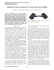one’s torso with one’s ankles to move the wheelsets. The mechanical model (Fig. 2) has a rotor situated at the center of the longitudinal axis to simulate the human rider’s torso. The rotor and whe...

### Multi-scale Feature Extraction for 3D Models using Local Surface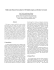### aspects regarding the signifiance of the curvature types and values### A Sparse Object Coding Scheme in Area V4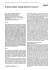### Position-time and velocity-time graphs Uniform### Joule`s Only General Exposition of the Principle of Conservation of### Britannica 15-th edition, 1991, Ether, Michelson A.A., Michelson### - Wiley Online Library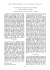### VIBRATION ANALYZER Vibration Analyzer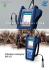### Gluhareff Pressure Jet Engine: Past, Present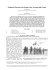### College Physics Chapter 2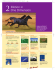### Groundkeeper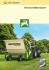### CCD Inspector and FWHM Monitor### Simson User Guide. Produced from Rev: 1053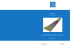### N8489B Gyrocopter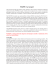### RANCANGAN PELAJARAN TAHUNAN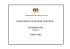### Oil Reclamation Services### Doppler weather radar in Australia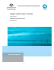### glas kurser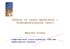### (LVCC) The Fifth - Corvair Society of America